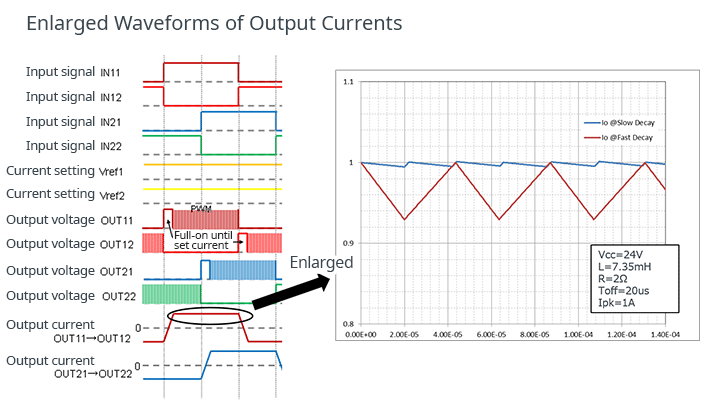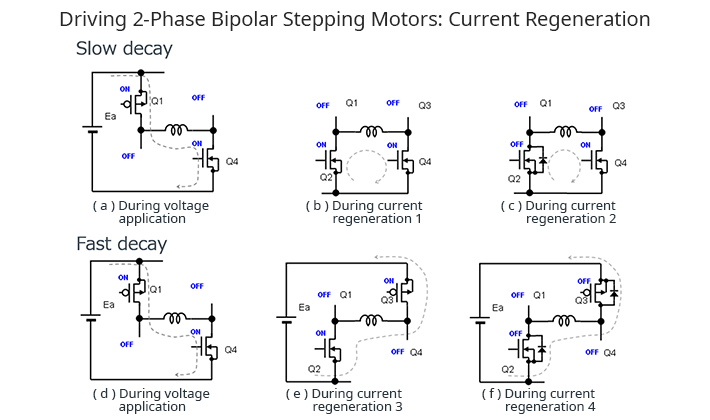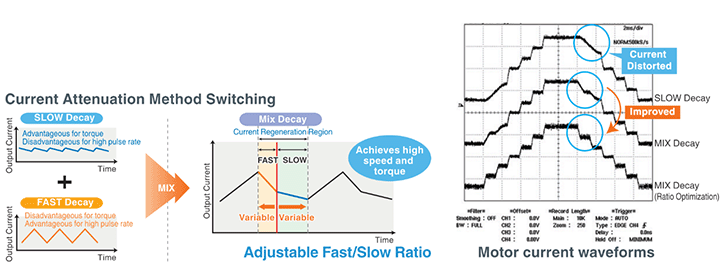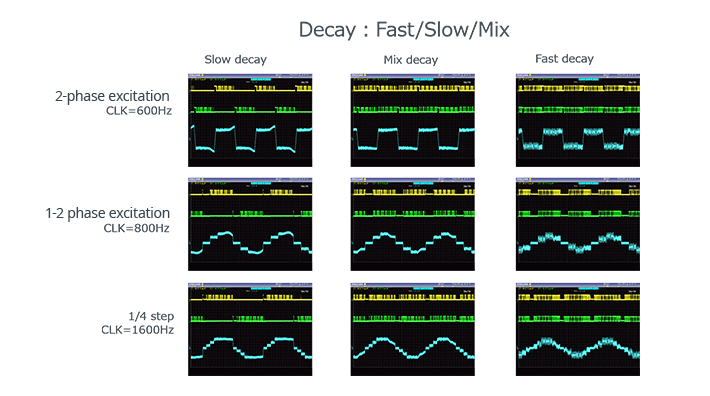# Driving 2-Phase Bipolar Stepping Motors: Part 2

2023.03.15

・Compared with Fast Decay, current ripples are smaller in Slow Decay, and so there is less noise. And the average current is greater; consequently the generated torque can be made larger. However, this is disadvantageous at fast pulse rates.

・Conversely, Fast Decay is disadvantageous with respect to noise and torque, but is advantageous for fast pulse rates.

・Mix Decay makes possible waveform optimization by combining Slow Decay and Fast Decay.

In “Driving 2-Phase Bipolar Stepping Motors: Part 1” in the previous article, an example of a basic driving circuit for a 2-phase bipolar stepping motor and driving waveforms were presented. This Part 2 explains decay, that is, current attenuation during current regeneration in driving of a 2-phase bipolar stepping motor.

## Driving 2-Phase Bipolar Stepping Motors: About Decay

In stepping motor driving, control over decay, which is current attenuation, is necessary. Decay is a method of causing current attenuation when the supply of current to the motor is turned off; there are essentially two methods, Slow Decay and Fast Decay.

Below is a part of the previously shown stepping motor driving waveforms. The output voltage OUT is a PWM signal, and so the output current is an average current that is turned on and off in concert with the PWM signal. Because it is coils that are doing the driving, the output current waveform is not a rectangular wave, as with the PWM voltage output, but a sawtooth wave. The diagram shows enlarged output current waveforms.The blue waveform is the Slow Decay waveform; the slope of the attenuation is gradual, so that the current attenuation rate is slow, and there is not much current attenuation during PWM off intervals. Hence the time until the set current is reached during on intervals is also short.

The red waveform is the Fast Decay waveform. The slope is steep, so that current attenuation is rapid, and the amount of attenuation is considerable; time is required during on intervals until the set current is reached, and so the period is longer than for Slow Decay.

## Slow Decay and Fast Decay: Differences in Methods

The decay method can be selected using the method of switching the H bridge on the driver side.The coil in the figure represents one coil among the coil sets A and B in a stepping motor. Among the four switches of the H bridge, the switches that are not parts of the current paths are omitted.

In (a) of the Slow Decay method, Q1 and Q4 are in the on state. In (b) and (c) Q4 is on, while Q2 is on and off respectively. The switch states differ, but in the turned-off Q2 MOSFET, regeneration current flows through the parasitic diode, and so current similarly flows in all of them. The only regeneration current that flows is what was stored in the coil.

In (d) using Fast Decay, the state is the same as for Slow Decay. In (e), Q2 and Q3 are turned on, and in (f), all the MOSFETs are turned off; regeneration current flows in the turned-on Q2 and Q3, and also flows in the turned-off Q2 and Q3 via the parasitic diodes, so that in both states, currents similarly flow. Currents flow toward the power supply, but because the power supply voltage acts to send current in the opposite direction, the current attenuates rapidly.

The Slow Decay and Fast Decay methods are used selectively according to the state in which to control turn-off.

## Differences in Decay Methods and the Relation to Stepping Motor Driving

In Slow Decay, current ripples are smaller compared with Fast Decay, so that there is less noise. And, the average current is greater, so that the generated torque can be increased. However, when the pulse rate is raised, waveform distortion occurs, and there is the drawback that the motor does not rotate properly.

Conversely, in the Fast Decay method there are disadvantages with respect to noise and torque, but the method has advantages over Slow Decay at high pulse rates.

The basic decay methods are Slow Decay and Fast Decay, but a Mix Decay method combines the two to utilize the advantages of both.

In Mix Decay, Fast Decay is used at the beginning of current attenuation, and thereafter Slow Decay is executed. As a result, attenuation is faster than when using only Slow Decay, and current ripples are suppressed compared with when using only Fast Decay. Mix Decay method can support high pulse rates while maintaining large torque. Some driver ICs enable adjustment of the time ratio of the Slow and Fast methods, so that the current waveform can be optimized.Below are the waveforms of Slow Decay, Mix Decay, and Fast Decay for each step number.. We see that Mix Decay results in an optimized waveform.